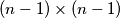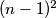### IMO Shortlist 1996 problem C2

Kvaliteta:
Avg: 0,0
Težina:
Avg: 6,0
A square$(n - 1) \times (n - 1)$ is divided into$(n - 1)^2$ unit squares in the usual manner. Each of the$n^2$ vertices of these squares is to be coloured red or blue. Find the number of different colourings such that each unit square has exactly two red vertices. (Two colouring schemse are regarded as different if at least one vertex is coloured differently in the two schemes.)
Izvor: Međunarodna matematička olimpijada, shortlist 1996